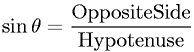Equations > Trigonometry > Trigonometric Definitions > Sine Definition for a Right Triangle

### Sine Definition for a Right TriangleLatex Code:

MathML Code:

 $\mathrm{sin}\theta =\frac\mathrm{Opposite}\mathrm{Side}\mathrm{Hypotenuse}$

MathType 5.0: